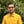Trusted answers to developer questions
Trusted Answers to Developer Questions

Related Tags

d
sqrt
math
communitycreator

# What is the sqrt() function in D?Harris Amjad

Grokking Modern System Design Interview for Engineers & Managers

Ace your System Design Interview and take your career to the next level. Learn to handle the design of applications like Netflix, Quora, Facebook, Uber, and many more in a 45-min interview. Learn the RESHADED framework for architecting web-scale applications by determining requirements, constraints, and assumptions before diving into a step-by-step design process.

The sqrt() function in D returns the square root of a non-negative number.

The figure below shows the mathematical representation of the sqrt() function:

Figure 1: Mathematical representation of the sqrt() function

Note: We need to import std.math in our code to use the sqrt() function. We can import it with the following:
import std.math

### Syntax

sqrt(number)
// The number should be floating, double, or real.


### Parameter

sqrt() requires a non-negative number as a parameter.

### Return value

sqrt() returns the square root of the number sent as a parameter.

Note:

• If -0.0 , real.infinity , real.nan, or -real.nan is sent as a parameter, the function returns the parameter as it is.
• If -real.infinity or number < 0.0 is sent as a parameter, the function returns -nan.

### Working example

The code below shows the use of the sqrt() function in D.

import core.stdc.stdio;import std.stdio;//Header required for the functionimport std.math;int main(){    //Perfect square root    writeln ("The value of sqrt(4.0) : ",sqrt(4.0));    writeln ("The value of sqrt(0.0) : ",sqrt(0.0));    writeln ("The value of sqrt(25.0) : ",sqrt(25.0));    //Non-perfect square root    writeln ("The value of sqrt(4.4) : ",sqrt(4.4));    //Exceptional outputs    writeln ("The value of sqrt(-0.0) : ",sqrt(-0.0));    writeln ("The value of sqrt(real.infinity) : ",sqrt(real.infinity));    writeln ("The value of sqrt(-real.infinity) : ",sqrt(-real.infinity));    writeln ("The value of sqrt(real.nan) : ",sqrt(real.nan));    writeln ("The value of sqrt(-real.nan) : ",sqrt(-real.nan));    writeln ("The value of sqrt(-4.5) : ",sqrt(-4.5));    return 0;}

### Explanation

• Line 4: We add the std.math header required for the sqrt() function.

• Lines 9 to 13: We calculate the square root value of the perfect square numbers 4, 0, and 25 using sqrt().

• Line 16: We calculate the square root value of a non-perfect square number 4.4 using sqrt().

• Lines 19 to 24: We calculate the square root value of exceptional numbers using sqrt().

RELATED TAGS

d
sqrt
math
communitycreator

CONTRIBUTORHarris Amjad

Grokking Modern System Design Interview for Engineers & Managers

Ace your System Design Interview and take your career to the next level. Learn to handle the design of applications like Netflix, Quora, Facebook, Uber, and many more in a 45-min interview. Learn the RESHADED framework for architecting web-scale applications by determining requirements, constraints, and assumptions before diving into a step-by-step design process.

Keep Exploring

Learn in-demand tech skills in half the time